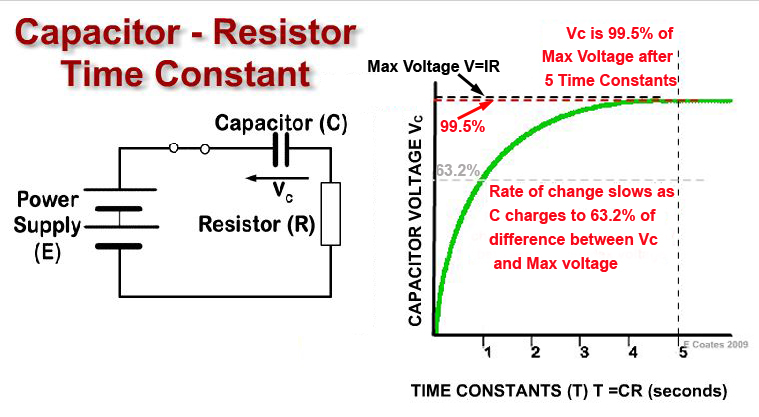# The CR Time Constant

• After studying this section, you should be able to describe:
• • The Time constant of a CR circuit
• ...and carry out calculations involving
• •Time constants in a simple CR circuit.### Fig. 4.3.1 The CR Time Constant.

When a voltage is applied to a capacitor it takes some amount of time for the voltage to increase. This increase happens in a curve that follows a mathematically "exponential" law to its maximum value, after which, the voltage will remain at this "steady state" value until there is some other external change to cause a change in voltage. From the instant the voltage is applied, the rate of change of the voltage is high, and if it was to continue in a linear manner, then VC would reach its maximum value in a time equal to one time constant (T), where T (in seconds) is equal to C (in Farads) multiplied by R (in ohms), see fig 4.3.1. above. That is: T = CR

## Fully Charged?

After about 5 time constant periods (5CR) the capacitor voltage will have very nearly reached the value E. Because the rate of charge is exponential, in each successive time constant period Vc rises to 63.2% of the difference in voltage between its present value, and the theoretical maximum voltage (VC = E). Therefore the 63.2% becomes a smaller and smaller voltage rise with each time constant period and although, for all practical purposes VC = E in fact VC never quite reaches the value of E.